Engineering Dynamics (EngM 373) -- Exam I -- Spring 1996

Problem # 1: A projectile is ejected into an experimental fluid at time t=0. The initial speed is vo and the angle to the horizontal is q. The drag on the projectile results in an acceleration term aD =-kv, where k is a constant and v is the velocity of the projectile. Determine the x- and y-components of both the velocity and displacement as a function of time. What is the terminal velocity? Include the effects of gravitational acceleration.

(10 points)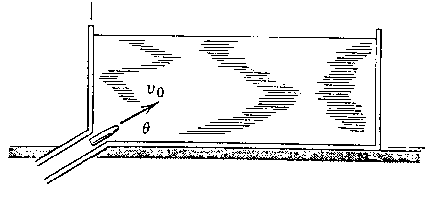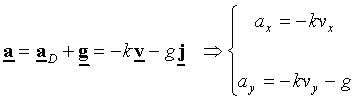x-component of velocity: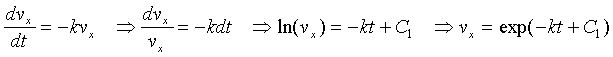Initial conditions: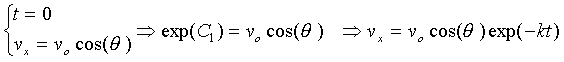x-component of position: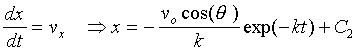Initial conditions: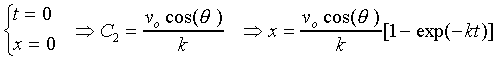y-component of velocity:Initial conditions: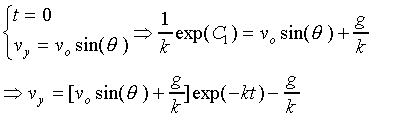y-component of position: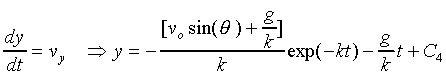Initial conditions: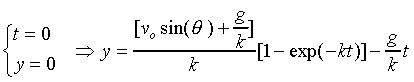Terminal velocity: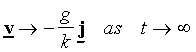Problem # 2: A rocket traveling above the atmosphere at an altitude of 500 km would have a free-fall acceleration g= 8.43 m/s2 in the absence of forces other than gravitational attraction. Because of thrust, however, the rocket has an additional acceleration component a1 of 8.8 m/s2 tangent to its trajectory, which makes an angle of 30o with the vertical at the instant considered. If the velocity v of the rocket is 30000 km/h at this position, compute the radius of curvature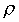of the trajectory and the rate at which v changes with time.

(10 points)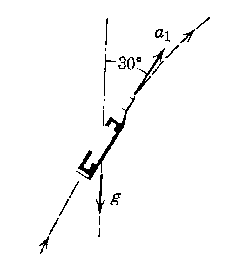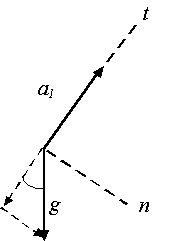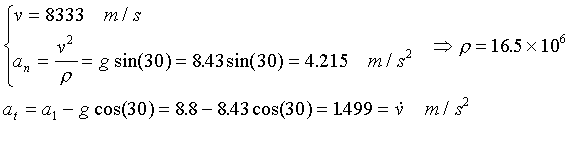Problem # 3: Collar A and B slide along the fixed rods and are connected by a cord of length L. If collar A has a velocity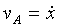to the right, express the velocity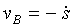of B in terms of x, vA, and s.

(10 points)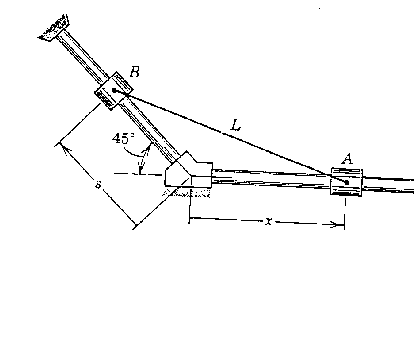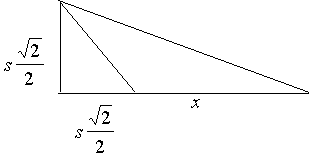For extra credit: If a point P is moving in the plane z=0, then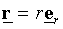, thus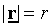. Why then isn't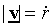.

(3 points)

For extra credit: show that for a vector b if |b| is constant, then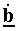is orthogonal to b.

(3 points)